# 3.1 经典二分问题

class Solution {
public int search(int[] nums, int target) {
//分别准备好左右端点
int left = 0, right = nums.length - 1;
//循环二分
while (left <= right) {
//取中点
int pivot = left + (right - left) / 2;
//找到答案并返回
if (nums[pivot] == target) return pivot;
//向左继续找
if (target < nums[pivot]) right = pivot - 1;
//向右继续找
else left = pivot + 1;
}
//未找到，返回-1
return -1;
}
}
class Solution:
def search(self, nums: List[int], target: int) -> int:
left, right = 0, len(nums) - 1
while left <= right:
pivot = left + (right - left) // 2
if nums[pivot] == target:
return pivot
if target < nums[pivot]:
right = pivot - 1
else:
left = pivot + 1
return -1
class Solution {
public:
int search(vector<int>& nums, int target) {
int pivot, left = 0, right = nums.size() - 1;
while (left <= right) {
pivot = left + (right - left) / 2;
if (nums[pivot] == target) return pivot;
if (target < nums[pivot]) right = pivot - 1;
else left = pivot + 1;
}
return -1;
}
};

# 3.2 基础二分小变形1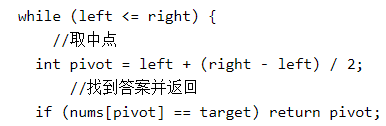public class Solution {
public int searchInsert(int[] nums, int target) {
int len = nums.length;
int left = 0;
int right = len - 1;
while (left <= right) {
int mid = (left + right) / 2;
if (nums[mid] < target) {
left = mid + 1;
} else {
right = mid - 1;
}
}
return left;
}
}

# 3.3基础二分小变形2

class Solution {
public int[] searchRange(int[] nums, int target) {
int[] ans=new int;
ans=searchRangeLeft(nums,target);
ans=searchRangeRight(nums,target);
return ans;
}
public int searchRangeLeft(int[] nums, int target) {
int left=0;
int right=nums.length-1;
while(left<=right){
int mid=(left+right)/2;
if(nums[mid]>target){
right=mid-1;
}else if(nums[mid]<target){
left=mid+1;
}else if(mid==0 || nums[mid-1]!=target){
return mid;
}else{
right=mid-1;
}
}
return -1;
}
public int searchRangeRight(int[] nums, int target) {
int left=0;
int right=nums.length-1;
while(left<=right){
int mid=(left+right)/2;
if(nums[mid]>target){
right=mid-1;
}else if(nums[mid]<target){
left=mid+1;
}else if(mid==nums.length-1 || nums[mid+1]!=target){
return mid;
}else{
left=mid+1;
}
}
return -1;
}
}

# 3.4泛化二分的概念while (范围没有缩小为0) {
if (满足某种条件) {
排除一半答案
} else {
排除另一半答案
}
}

-1 : 我的数字比较小
1 : 我的数字比较大
0 : 恭喜！你猜对了！

/* The guess API is defined in the parent class GuessGame.
@return -1 if my number is lower, 1 if my number is higher, otherwise return 0
int guess(int num); */

public class Solution extends GuessGame {
public int guessNumber(int n) {
int low = 1;
int high = n;
while (low <= high) {
int mid = low + (high - low) / 2;
int res = guess(mid);
if (res == 0)
return mid;
else if (res < 0)
high = mid - 1;
else
low = mid + 1;
}
return -1;
}
}

# 3.5在线性结构上二分的题目积累

或者返回索引 5， 其峰值元素为 6。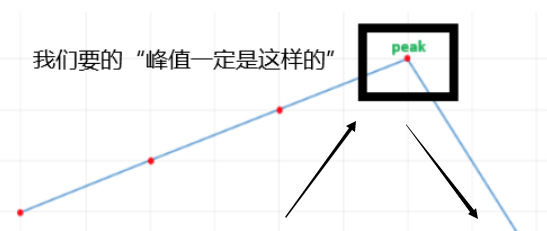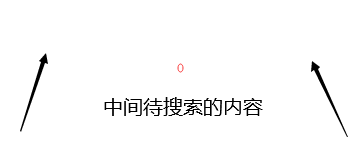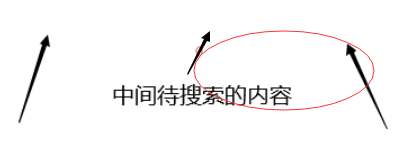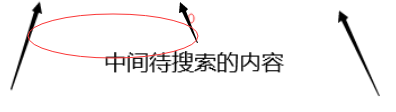public class Solution {
public int findPeakElement(int[] nums) {
int l = 0, r = nums.length - 1;
while (l < r) {
int mid = (l + r) / 2;
if (nums[mid] > nums[mid + 1])
r = mid;
else
l = mid + 1;
}
return l;
}
}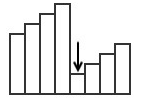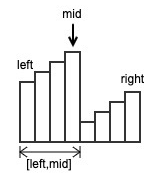[left,right] 表示当前搜索的区间。

class Solution {
public int findMin(int[] nums) {
if(nums.length==1)return nums;
if(nums<nums[nums.length-1])return nums;
int left=0;
int right=nums.length-1;
int mid=0;
while(left<right){
mid=(left+right)/2;
if(nums[mid]>=nums){
left=mid+1;
}else{
right=mid;
}
}
return nums[right];
}
}

# 3.6二维数组的二分查找

matrix = [
[1,   3,  5,  7],
[10, 11, 16, 20],
[23, 30, 34, 50]
]
target = 3

matrix = [
[1,   3,  5,  7],
[10, 11, 16, 20],
[23, 30, 34, 50]
]
target = 13

class Solution {
public boolean searchMatrix(int[][] matrix, int target) {
if (matrix == null || matrix.length == 0) {
return false;
}
int row = matrix.length;
int col = matrix.length;
int start = 0;
int end = row * col - 1;
while (start <= end) {
int mid = start + (end - start) / 2;
if (matrix[mid / col][mid % col] == target)return true;
else if (matrix[mid / col][mid % col] > target)end = mid - 1;
else start = mid + 1;
}
return false;
}
}

# 3.7二叉树上二分的题目积累

4
/ \
2   5
/ \
1   3

/**
* Definition for a binary tree node.
* public class TreeNode {
*     int val;
*     TreeNode left;
*     TreeNode right;
*     TreeNode(int x) { val = x; }
* }
*/
class Solution {
public int closestValue(TreeNode root, double target) {
int val, closest = root.val;
while (root != null) {
val = root.val;
closest = Math.abs(val - target) < Math.abs(closest - target) ? val : closest;
root =  target < root.val ? root.left : root.right;
}
return closest;
}
}

1
/ \
2   3
/ \  /
4  5 6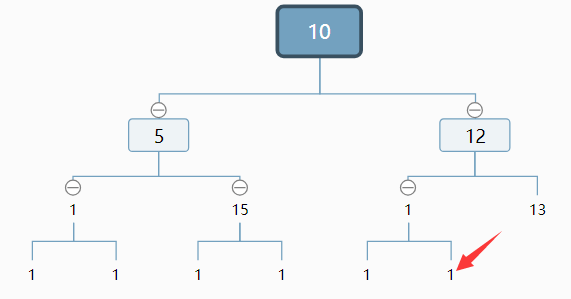1）找到右子树的最左结点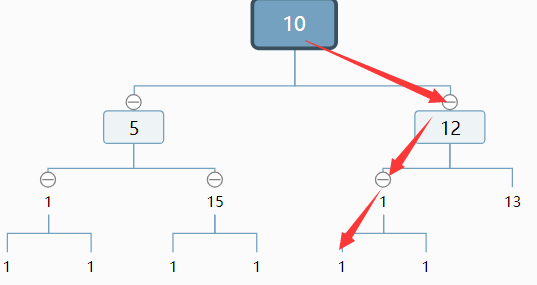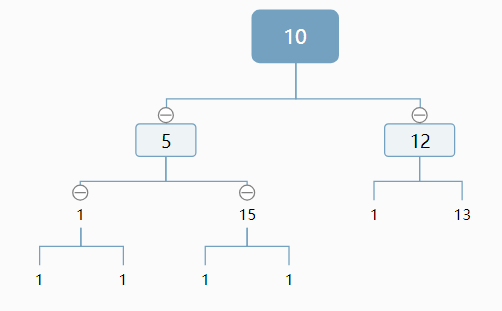2）判断之后，如果是这种情况，我们排除了左子树，计算排除的结点个数，并对右子树（如图2）做相同的处理。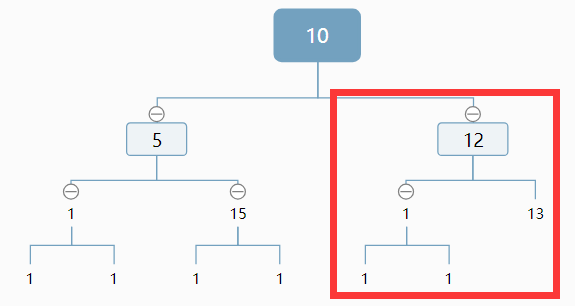3）对方框内重复此过程。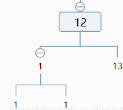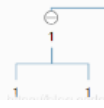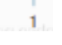最终找到它。

​
public class Demo {
public static class Node {
public int value;
public Node left;
public Node right;

public Node(int data) {
this.value = data;
}
}
//返回结点个数
public static int nodeNum(Node head) {
return 0;
}
}
//返回根为node，当前层数为l，总深度为h的结点个数
public static int bs(Node node, int l, int h) {
if (l == h) {
return 1;
}
if (mostLeftLevel(node.right, l + 1) == h) {             //右子树最深一行最左为空
return (1 << (h - l)) + bs(node.right, l + 1, h);    //右bs+左子树结点个数
} else {                                                 //右子树最深一行最左不为空
return (1 << (h - l - 1)) + bs(node.left, l + 1, h);//左bs+右子树结点个数
}
}
//计算树的高度
public static int mostLeftLevel(Node node, int level) {
while (node != null) {
level++;
node = node.left;
}
return level - 1;
}

public static void main(String[] args) {
}
}
​

我们再回忆第4节泛化概念时给的伪代码：

while (范围没有缩小为0) {
if (满足某种条件) {
排除一半答案
} else {
排除另一半答案
}
}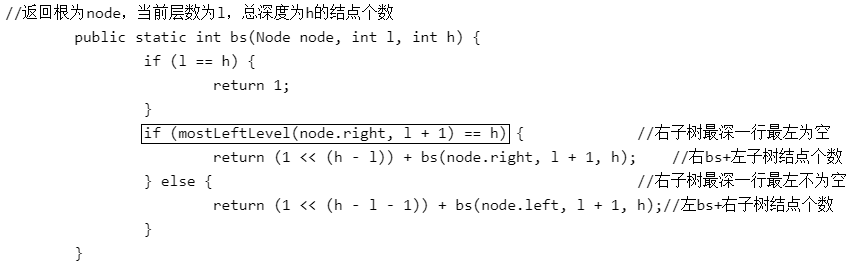# 3.8数学中的二分

由于返回类型是整数，小数部分将被舍去。

public class Solution {

public int mySqrt(int x) {
long left = 0;
long right = Integer.MAX_VALUE;
while (left < right) {
long mid = (left + right + 1) >>> 1;
long square = mid * mid;
if (square > x) {
right = mid - 1;
} else {
left = mid;
}
}
return (int) left;
}
}

# 3.9最大化或最小化问题

#include <cstdio>
#include <cmath>
using namespace std;
const int M=10005;
const double inf=200005.0;
double L[M];
int n,k;
bool judge(double x)//判断解是否可行
{
int num=0;
for(int i=0;i<n;i++)num+=(int)(L[i]/x);
return num>=k;
}
void solve()//二分答案
{
double left=0,right=inf;
for(int i=0;i<100;i++) //代替while(r>l) 避免了精度问题
{ //1次循环可以把区间缩小一半，100次可以达到10^(-30)的精度
double mid=(left+right)/2;
if(judge(mid)) left=mid;
else right=mid;
}
printf("%.2f\n",floor(right*100)/100);
}
int main()
{
while(scanf("%d%d",&n,&k)!=-1)
{
for(int i=0;i<n;i++)scanf("%lf",&L[i]);
solve();
}
}

POJ2456

# 3.10总结

10-303509-07481
10-095601
07-021879
05-11175
04-0430
02-271653
04-0194
02-041758